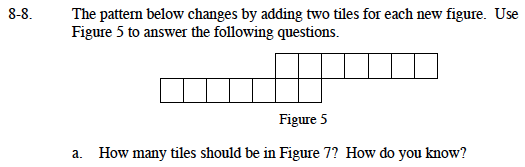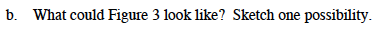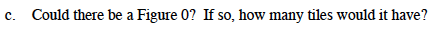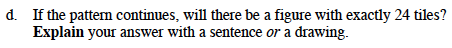### Home > MC1 > Chapter 8 > Lesson 8.1.1 > Problem8-8

8-8.How many tiles are in figure 5? If you add two tiles for Figure 6 and two more for Figure 7, how many tiles should be in Figure 7?If there are 14 tiles in Figure 5, and you take away two for Figure 4 and two more for Figure 3, how many tiles should be in Figure 3?Yes! If you have 14 tiles and take two away five times, how many tiles should Figure 0 have?If Figure 0 has 4 tiles, how many times do you need to add two tiles to get to 24 tiles?